Name:    MCAS Heat and Temperature

Multiple Choice
Identify the choice that best completes the statement or answers the question.

1.

A party shop delivers helium-filled balloons to homes and businesses. The owners realize from experience that on hot summer days they should inflate the balloons only three-quarters full. On cold winter days they can fully inflate the balloons. Which of the following is the best hypothesis to explain this observation? (MCAS 2004)
 a. The helium gas is more active in the winter season. b. Air outside the balloons leaks into the balloons. c. As the temperature increases, the helium in the balloons expands. d. Outdoor air pressure in the summer is less than indoor air pressure.

2.

Perfume sprayed from a bottle spreads more easily in a warm room of 25°C than in a cool room of 15°C. Which of the following correctly compares perfume molecules at 25°C to those at 15°C? (MCAS 2006)
 a. At 25°C, they have more mass. b. At 25°C, they are moving faster. c. At 25°C, they have less kinetic energy. d. At 25°C, they are decreasing in volume.

3.

A recycling plant manager needs to melt 1500 kg of scrap copper to sell to a wire manufacturer. The copper is at 15°C and its melting point is 1083°C. The copper has a specific heat of 385 J/kg • K. How much heat is required to raise the temperature of the copper to its melting point? (MCAS 2005)
 a. 6.2 ´ 108 J b. 6.3 ´ 108 J c. 7.7 ´ 108 J d. 7.9 ´ 108 J

4.

Two boxes, A and B, both contain the same number of nitrogen gas molecules.
The gas molecules in box A have twice the average speed of the molecules in box B. Which of the following best describes the nitrogen gas in box A? (MCAS 2005)
 a. The nitrogen gas in box A has a greater mass than the nitrogen gas in box B. b. The nitrogen gas in box A has a greater density than the nitrogen gas in box B. c. The nitrogen gas in box A has a greater temperature than the nitrogen gas in box B. d. The nitrogen gas in box A has a greater specific gravity than the nitrogen gas in box B.

5.

A 10 g sample of aluminum and a 10 g sample of iron were each heated by 100 joules of energy. The temperature of the aluminum sample rose 11°C, while the temperature of the iron sample increased 23°C. Which statement best accounts for these results? (MCAS 2005)
 a. Iron is twice as dense as aluminum. b. Atoms of aluminum are smaller than atoms of iron. c. Using equal masses results in similar heat capacities. d. The specific heat of iron is less than that of aluminum.

6.

In a copper wire, a temperature increase is the result of which of the following? (MCAS 2006)
 a. an increase in the size of the copper particles b. a decrease in the mass of the copper particles c. an increase in the motion of the copper particles d. a decrease in the distance between the copper particles

7.

The masses and specific heats of some samples of liquids are shown in the table below.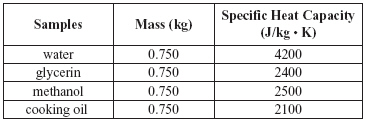The temperature of which sample will rise most when 1000 J of heat is added? (MCAS 2006)
 a. water b. glycerin c. methanol d. cooking oil

8.

The illustration below shows a student bending a piece of wire back and forth at a single point X. The wire’s temperature rises noticeably at point X.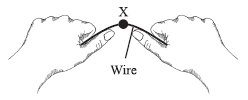Which of the following best describes the source of the temperature increase? (MCAS 2006)
 a. Some of the wire’s mass is transformed into heat energy as the wire is bent. b. Some of the kinetic energy is transformed into heat as the wire is bent. c. The bending transfers potential energy to the wire, heating it. d. The bending causes a current that heats the wire.

9.

The diagram below represents four empty copper containers at room temperature.An equal amount of water at 90°C is added to each copper container. Assume there is no loss of heat to the environment. Which container will have had the greatest change in temperature when the water and the container reach equilibrium? (MCAS 2006)
 a. 1 b. 2 c. 3 d. 4

10.

The water contained in a geyser system gains energy from the underground material surrounding it. The water molecules gain kinetic energy and this results in an increase in the pressure of the water. Eventually the geyser erupts and expels water into the air above ground. Which of the following types of energy is the source for the initial energy gain of the water? (MCAS 2006)
 a. electrical b. magnetic c. mechanical d. thermal

11.

Which of the following changes occurs as a solid is heated?
 a. The kinetic energy of the solid decreases. b. The average density of the solid increases. c. The specific heat capacity of the solid decreases. d. The average molecular speed in the solid increases.

12.

The instructions below outline the procedure for a demonstration.

Materials:
four 100 g metal blocks, each of a different metal
four polystyrene foam cups, each containing 150 g of 10°C water

Procedure:
1. Place the four cups of water next to each other on the lab bench.
2. Heat each block to a temperature of 60°C.
3. Place each heated block in a separate cup of 10°C water.
4. Measure the temperature change in each cup of water after 100 s.

At the end of the demonstration, which block raised the temperature of the 10°C water the greatest amount? (MCAS 2006)
 a. the block with the greatest density b. the block with the lowest surface area c. the block with the greatest specific heat d. the block with the lowest thermal conductivity

13.

The graph below represents changes in molecular motion in a solid plastic cylinder over time.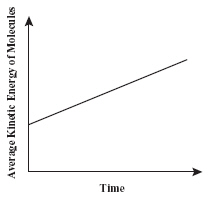These changes in the molecules of the plastic cylinder must be accompanied by which of the following? (MCAS 2006)
 a. an increase in mass c. an increase in temperature b. a decrease in volume d. a decrease in heat capacity

14.

Thermal energy is added to four identical 1.0 kg samples of water at room temperature.Which of the following increases in each sample? (MCAS 2007)
 a. average charge of an electron c. average mass of a proton b. average density of a nucleus d. average speed of a molecule

15.

To make ice, water must first be cooled. The specific heat of water is 4,186 J/kg · °C. Approximately how much heat must be removed from 0.50 kg of water to change its temperature from 24°C to 5°C? (MCAS 2007)
 a. 0 J b. 19,900 J c. 39,800 J d. 79,500 J

16.

A rise in the temperature inside a kitchen means there is an increase in the (MCAS 2007)
 a. density of the air molecules. b. number of the air molecules. c. average size of the air molecules. d. average kinetic energy of the air molecules.

17.

Jessica places 1000 g of water at 20.0°C in an insulated cup. The specific heat
capacity of water is 4.20 J/g · °C. What is the final temperature of the water if 5000 J of energy is added to the water in the insulated cup? (MCAS 2007)
 a. 20.1°C b. 20.5°C c. 21.2°C d. 25.0°C

18.

Two identical beakers each contain 250 mL of water. The temperature of the water is 85°C in one beaker and 15°C in the other beaker. A drop of red food coloring is placed in each beaker at the same time. During the first minute, which of the following is most likely to happen? (MCAS 2007)
 a. The food coloring will spread out faster in the 85°C water than in the 15°C water. b. The food coloring will form a layer at the top of each beaker since it does not mix with water. c. The food coloring will spread only halfway through the water in each beaker due to convection currents. d. The food coloring will settle faster at the bottom of the beaker containing 85°C water than at the bottom of the beaker containing 15°C water.

19.

Which of the following requires the greatest amount of heat? (MCAS 2007)
 a. increasing the temperature of 1 kg of water from 0°C to 30°C b. increasing the temperature of 10 kg of water from 10°C to 20°C c. increasing the temperature of 20 kg of water from 19°C to 21°C d. increasing the temperature of 50 kg of water from 20°C to 21°C

20.

If heat is added to a liquid, which of the following occurs? (MCAS 2007)
 a. The friction in the liquid increases. b. The size of the liquid molecules expands. c. The potential energy of the liquid changes. d. The molecular motion in the liquid increases.

21.

A sealed glass container holds molecules of nitrogen gas. The nitrogen molecules
have increasing velocities.Which of the following will make the molecules move faster? (MCAS 2007)
 a. bonding the molecules together c. expanding the volume of the container b. transferring heat to the molecules d. decreasing the pressure in the container

22.

A recycling plant manager needs to melt 1500 kg of scrap copper to sell to a wire manufacturer. The copper is at 15°C and its melting point is 1083°C. The copper has a specific heat of 385 J/kg·K. How much heat is required to raise the temperature of the copper to its melting point? (MCAS 2008)
 a. 6.2 ´ 108J b. 6.3 ´ 108J c. 7.7 ´ 108J d. 7.9 ´ 108J

23.

Measurements inside a sealed container show that the pressure exerted by a gas increases as the thermal energy of the gas increases. Which of the following best explains this pressure increase? (MCAS 2008)
 a. The gas molecules stick to the walls of the container. b. The gas molecules radiate energy in the form of nuclear particles. c. The gas molecules begin to bond together into heavier molecules. d. The gas molecules move faster and strike the container walls more frequently.

24.

A heated rock is placed in a container of water that is cooler than room temperature. Which of the following statements best describes what happens? (MCAS 2008)
 a. Cold is removed from the container of water until the rock, the container, and the water all reach the same final temperature. b. The heated rock loses heat to the container of water until the rock, the container, and the water all reach the same final temperature. c. The heated rock loses heat to the container of water until the rock, the container, and the water each reach a different final temperature. d. Cold is removed from the container of water until the rock, the container, and the water each reach a final temperature lower than their original temperatures.

25.

A student hypothesizes that the mass of a substance affects how the temperature of the substance changes when it is heated. The student uses the following procedure to test the hypothesis.

· Each sample is initially at room temperature before heating.
· Each sample is heated for the same amount of time with the same heat source.
· The final temperature is measured for each sample.

Which of the following would be the best way to select the samples for testing the student’s hypothesis? (MCAS 2008)
 a. Obtain samples of one substance, each with the same mass. b. Obtain samples of one substance, each with a different mass. c. Obtain samples of different substances, each with the same mass. d. Obtain samples of different substances, each with a different mass.

26.

A window washer noticed that, from the same distance and with no breeze, the smell of ammonia glass cleaner reached him faster on a hot day (30°C) than on a cold day (5°C). Which of the following explains this observation? (MCAS 2008)
 a. Molecules expand at higher temperatures. b. Molecules move more rapidly at higher temperatures. c. The convection currents carry molecules at higher temperatures. d. The chemical reaction of molecules increases at higher temperatures.

27.

Which of the following statements best explains why there is no change in temperature once thermal equilibrium between two objects in contact is reached? (MCAS 2009)
 a. Heat can flow between two objects in contact. b. Heat does not flow between objects in contact at the same temperature. c. Heat does not flow spontaneously from a cooler object to a warmer object. d. Heat flows by conduction, convection, and radiation when the temperatures are the same.

28.

The table below shows data from a heating experiment.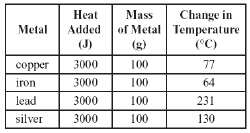Which of the following conclusions is supported by the data in the table? (MCAS 2009)
 a. A given mass of silver requires less heat to change its temperature 1°C than an equal mass of iron. b. A given mass of silver requires less heat to change its temperature 1°C than an equal mass of lead. c. A given mass of copper requires less heat to change its temperature 1°C than an equal mass of lead. d. A given mass of copper requires less heat to change its temperature 1°C than an equal mass of silver.

29.

Which of the following statements best explains why drops of liquid form on the outside of a glass of ice water on a hot day? (MCAS 2009)
 a. Water molecules in the air are attracted to the surface of the glass. b. Water molecules in the ice water lose energy as they transfer heat to the air. c. Water molecules in the air lose energy as they transfer heat to the cold glass. d. Water molecules in the ice water move through the glass pores to the outside of the glass.

30.

The figure below represents water in a flask being heated by a hot plate.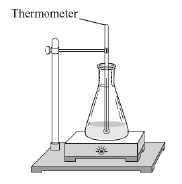Which of the following actions will slow down the rate of temperature increase of the water in the flask? (MCAS 2009)
 a. covering the flask with a piece of foil c. increasing the temperature of the hot plate b. adding more water at room temperature d. reducing the amount of water being heated

31.

A balloon is inflated with room-temperature air and then put in a sunny place. The balloon expands slightly due to a rise in temperature. Which of the following best describes the molecules of air inside the balloon when the balloon is left in the sunlight as compared to when it was first inflated? (MCAS 2010)
 a. The molecules are moving faster. b. There are more molecules in the balloon. c. There are fewer interactions between the molecules. d. The molecules stop colliding with the walls of the balloon.

32.

The specific heat of wood is about 1,700 J/ kg•°C. How much energy is required to heat a 12 kg piece of wood from 20°C to 30°C? (MCAS 2010)
 a. 1,400 J b. 17,000 J c. 204,000 J d. 612,000 J

33.

Two full 2 L bottles of water are placed on a table. Bottle 1 was just removed from a refrigerator; bottle 2 is at room temperature. Which of the following statements describes the average molecular kinetic energy and the speed of the molecules in each bottle? (MCAS 2010)
 a. Bottle 1 has the same average molecular kinetic energy as bottle 2, and the molecules have the same average speed in both bottles. b. Bottle 1 has a smaller average molecular kinetic energy than bottle 2, and the molecules have the same average speed in both bottles. c. Bottle 1 has a smaller average molecular kinetic energy than bottle 2, and the molecules have a slower average speed in bottle 1 than in bottle 2. d. Bottle 1 has a larger average molecular kinetic energy than bottle 2, and the molecules have a slower average speed in bottle 1 than in bottle 2.

34.

The molecules in which of the following have the greatest average molecular kinetic energy? (MCAS 2011)
 a. air in a person’s lungs c. raindrops collecting in a puddle b. ice cubes in a glass of iced tea d. water boiling in a pot on a stove

35.

Two blocks of zinc with different masses are at room temperature. Each block then absorbs the same amount of heat over 10 minutes. When the temperature of each block is then measured, block 1 is at a higher temperature than block 2. Which of the following statements most likely describes the zinc blocks? (MCAS 2011)
 a. The mass of block 1 is less than the mass of block 2. b. The mass of block 1 is greater than the mass of block 2. c. The specific heat of block 1 is less than the specific heat ofblock 2. d. The specific heat of block 1 is greater than the specific heat of block 2.

36.

A large container of cold water is moved from a refrigerator to a table. A hot piece of metal is placed into the water. Which of the following best describes the final temperature of the metal? (MCAS 2011)
 a. higher than the final temperature of the water b. the same as the final temperature of the water c. lower than the initial temperature of the water d. the same as the initial temperature of the water

37.

A student touches a metal sink with her finger and notices that her finger feels cold. Which of the following statements explains this observation? (MCAS 2011)
 a. Heat energy flows from the student’s finger to the metal sink. b. Heat energy flows from the metal sink to the student’s finger. c. The student’s finger was originally at the same temperature as the sink. d. The student’s finger was originally at a lower temperature than the sink.

38.

A cup of tea with a temperature of 80°C is placed on a table in a 20°C room. What happens to the thermal energy and molecular motion of the tea when it is left on the table? (MCAS 2012)
 a. The thermal energy increases and the molecules move faster. b. The thermal energy decreases and the molecules move faster. c. The thermal energy increases and the molecules move more slowly. d. The thermal energy decreases and the molecules move more slowly.

39.

A small body of water contains 2,000 kg of water. The specific heat of water is 4,180 J/kg°C. If the temperature of the water changes from 25°C to 20°C, approximately how much heat moves from the water to the surroundings? (MCAS 2012)
 a. 8,400,000 J b. 42,000,000 J c. 168,000,000 J d. 210,000,000 J

40.

A student slowly heats a beaker of a liquid on a hot plate. The liquid has a boiling point of 78°C. The student makes the graph shown below from the data she records as the liquid is heating.Which of the following statements best describes what happens to the molecules of liquid between 5 and 10 minutes of heating? (MCAs 2013)
 a. The mass of the molecules increases. b. The molecules undergo a chemical change. c. The molecules absorb energy to change phase. d. The average kinetic energy of the molecules decreases.

41.

Which of the following substances has the lowest average molecular kinetic energy? (MCAS 2013)
 a. nitrogen at 75°C b. quartz at 25°C c. steel at 50°C d. water at 10°C

42.

Calorimeters are instruments used to measure heat. The diagrams below show a calorimeter before a metal sample is added and after the sample is added.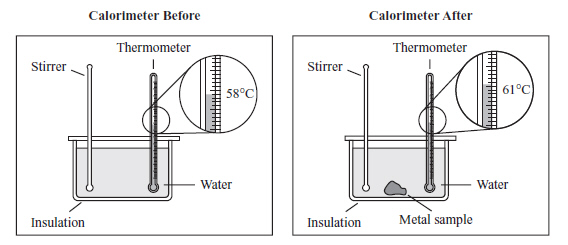Which of the following statements describes the flow of heat energy after the metal sample is added? (MCAS 2013)
 a. Heat energy flows from the stirrer to the thermometer. b. Heat energy flows from the water to the metal sample. c. Heat energy flows from the metal sample to the water. d. Heat energy flows from the insulation to the thermometer.

43.

A container of water with an initial temperature of 90°C is placed in a room where the temperature is 20°C. Which of the following statements describes what happens to the water over the next several hours? (MCAS 2014)
 a. The thermal energy of the water decreases, and its temperature decreases to 5°C. b. The thermal energy of the water decreases, and its temperature decreases to 20°C. c. The thermal energy of the water remains constant, and its temperature decreases to 55°C. d. The thermal energy of the water remains constant, and its temperature decreases to 70°C.

44.

The graph below shows the temperature of a sample of water as it is heated at a constant rate.Which of the following conclusions is best supported by the information in the graph? (MCAS 2014)
 a. The water boils between points X and Y and condenses between points Y and Z. b. The average kinetic energy of the water molecules increases between points X and Y and stays the same between points Y and Z. c. The water evaporates between points X and Y and the average kinetic energy of the water molecules increases between points Y and Z. d. The average kinetic energy of the water molecules remains the same between points X and Y and the water boils between points Y and Z.

45.

Thermal energy is added to a sample of 15°C water. Which of the following quantities must increase? (MCAS 2014)
 a. the water’s mass c. the water’s specific heat b. the water’s salinity d. the water’s temperature

46.

The illustration below represents an experiment in which a hot object is added to a container of water at room temperature.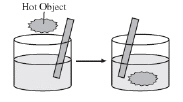The water is continuously stirred while the hot object is immersed in it. Which of the following graphs best shows the temperature changes that follow? (MCAS 2005)
 a.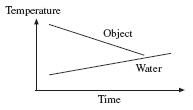c.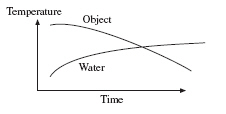b.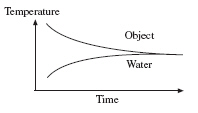d.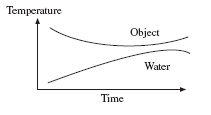47.

Which of the following figures correctly shows the conduction of heat within the system of metal blocks? (MCAS 2005)
 a.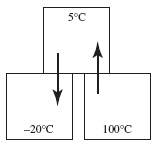c.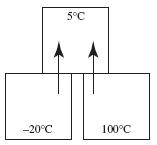b.d.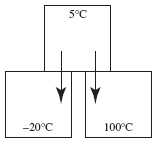48.

When one end of a short metal bar is heated, the opposite end will eventually become hot. Which of the following processes transfers the heat through the bar? (MCAS 2007)
 a. condensation b. conduction c. convection d. radiation

49.

A student places four identically sized metal blocks on a hot plate at 80°C. Then the student places an identical piece of wax on top of each block, as shown in the diagram below.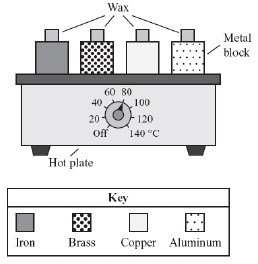The student measures the time it takes for the piece of wax on each block to melt. Which of the following questions is being studied in this experiment? (MCAS 2009)
 a. What is the melting point of the wax? c. Which metal radiates heat the best? b. What is the melting point of each metal? d. Which metal conducts heat the best?

50.

Sunlight heats a dark rock. A snake then moves onto the rock in order to increase its body temperature. Which of the following sequences represents how heat energy is transferred from the Sun to the rock to the snake? (MCAS 2014)
 a. radiation then conduction c. conduction then radiation b. radiation then convection d. convection then radiation

51.

Which of the following statements describes an advantage of using a wooden spoon instead of a metal spoon to stir a pot of hot soup? (MCAS 2014)
 a. A wooden spoon radiates heat faster than a metal spoon. b. A wooden spoon has a lower specific heat than a metal spoon. c. A wooden spoon does not conduct heat to its handle as quickly as a metal spoon. d. A wooden spoon lowers the boiling point of the soup, but a metal spoon does not.

52.

What method of heat transfer allows the Sun’s heat energy to reach Earth through the vacuum of space? (MCAS 2013)
 a. condensation b. conduction c. convection d. radiation

53.

A cup containing 25 mL of hot water and a similar cup containing 25 mL of cold water are placed on a table in a room at 21°C. Which of the following graphs shows the most likely change in temperature for each cup from 0 min to 40 min? (MCAS 2010)
 a.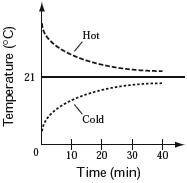c.b.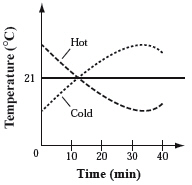d.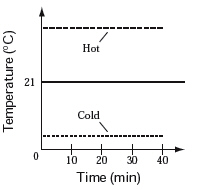54.

Which of the following is an example of heat transfer by conduction? (MCAS 2010)
 a. sunlight heating a floor b. an electric stove heating an iron pan c. a wood stove heating nearby objects through electromagnetic waves d. an electric heater heating air, which rises and is replaced with cooler air

55.

In which of the following examples is heat transferred primarily by radiation? (MCAS 2011)
 a. A fan blows warm air arounda room. b. A hot water bottle warms aperson’s feet. c. Water vapor from a shower warms the room. d. A spoon held near the side of a flame gets warm.

56.

Which of the following statements best explains how heat flows by conduction? (MCAS 2013)
 a. A large mass of air begins to move faster. b. Energy is transferred by electromagnetic waves. c. Energy is transferred from molecule to molecule. d. A large mass of warm air rises, replacing the cooler air above.

57.

An iron bar is placed in a flame, as shown below, and is heated until theend glows.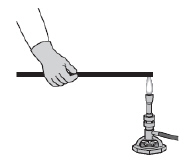The other end of the iron bar soon becomes hot, too. Which of the following statements best describes what happens? (MCAS 2012)
 a. A convective flow of energy is set up inside the iron bar. b. Energy is conducted from atom to atom along the length of the iron bar. c. Radiation moves through the iron bar and is absorbed by the end not in the flame. d. Air heated by the flame radiates down the iron bar and heats the end not in the flame.

58.

A sealed glass jar containing 200 mL of cold water is lowered into a large beaker containing 200 mL of hot water. Which of the following graphs shows how the temperature of each water sample will most likely change over time? (MCAS 2012)
 a.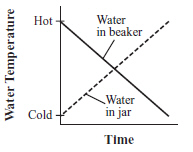c.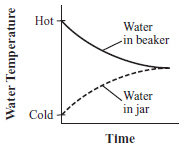b.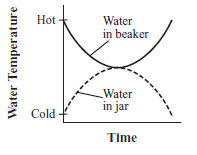d.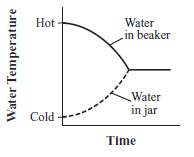59.

Popcorn is made by heating corn kernels. Different methods may be used to heat the kernels.
Which of the following methods uses radiation as the primary means of transferring energy to the corn kernels? (MCAS 2012)
 a. heating corn kernels in a hot air popper b. heating corn kernels in a microwave oven c. heating corn kernels in a foil pan on a hot plate d. heating corn kernels in oil in a pot on an electric stove

Open Response

60.

The table below shows initial data from a laboratory demonstration.In the demonstration, a heated 0.06 kg copper pipe was dropped into a beaker of water. Students in the class were asked to predict the final temperature of the beaker of water when it reached equilibrium with the copper pipe. (MCAS 2004)

a.       If this demonstration took place in a closed insulated beaker, instead of in an open beaker, what would be the relationship between the heat lost by the copper and the heat gained by the water and glass?

b.       Which material in the table above requires the most heat for a given change in temperature?

c.       The students found that the measured temperature was lower than they predicted. How would the students best explain this difference from the predicted temperature?

d.       Assume a heated 0.12 kg copper pipe was used instead of the given pipe. How would this change affect the final temperatures of the water and of the glass beaker?

61.

On a hot day, a student places a glass of cold lemonade on a table outdoors. After a few minutes, water droplets have formed on the outside of the glass. (MCAS 2011)

a.       Is energy absorbed or released by the cold lemonade? Explain your answer.

b.       Compare average kinetic energy for the air molecules and lemonade moleculeswhen the student first places the lemonade outdoors. Explain your answer.

c.       Explain how and why the water droplets form on the outside of the glass.

62.

The illustration below shows a container of water on an electric hot plate. Point A is in the water close to the hot plate, and point B is in the water near the top of the container.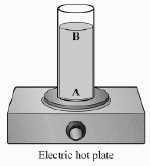The water in the container is at room temperature before the hot plate is turned on. (MCAS 2006)

a.       Describe the differences in the average motion of the water molecules at point A and at point B shortly after the hot plate is turned on.

b.       The water is heated until a thermometer placed in the center of the container reaches 100°C. Compare the average motion of the water molecules at points A and B at this temperature and explain your answer.

c.       The hot plate is then turned off. Describe the average motion of the molecules at points A and B after several hours.

63.

In an experiment, liquid 1 is at room temperature (21°C) and liquid 2 has been heated until it is warmer than room temperature. A student takes equal amounts of liquid 1 and liquid 2 and mixes them together in a beaker. (MCAS 2008)

a.       Compare the initial temperatures of liquid 1 and liquid 2 with the temperature of the mixture in the beaker at each of the following times:

· 5 minutes after mixing

· 2 hours after mixing

b. Describe the transfer of heat energy between the two liquids in the beaker.

c. Identify one other possible heat energy transfer in this experiment.

64.

In a room at 21°C, 60 mL of water is poured into each of three cups. The water in cup 1 is at 15°C, the water in cup 2 is at 25°C, and the water in cup 3 is at 45°C. (MCAS 2009)

a.       Describe what happens to the temperature of the water in each cup over a one-hour period.

b.       Describe what happens to the energy present in each cup over the one-hour period and explain why.

c.       Explain how equilibrium is reached in the cups.

65.

A car’s brakes absorb a large amount of energy and heat up as the car slows down and stops. Several materials with different thermal properties have been used for car brakes. In the table below, the specific heat capacities of three of these materials are shown. (MCAS 2010)In an experiment, 500 g each of aluminum, carbon-ceramic, and steel are heated and their temperature changes are recorded. The amount of heat absorbed and the temperature change for the 500 g sample of steel are shown in the graph below.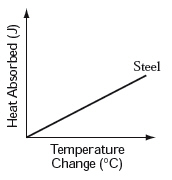a.       Draw a line on the graph to show the relationship expected for the 500 g sample of aluminum. Draw a line on your graph to show the relationship expected for the 500 g sample of carbon-ceramic. Identify each line on your graph, and explain why you drew each line where you did.

b.       If brake materials get too hot they soften or melt, resulting in loss of braking power. Of the three materials in the table, which is best suited to resist large changes in temperature? Explain your answer.

66.

A can of juice at 20°C is completely submerged in a closed, insulated container filled with water at 4°C, as shown in the diagram below. (MCAS 2012)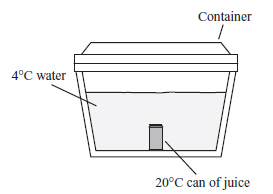a. Describe what happens to the temperature of the can of juice and the temperature of the water in the container within the first few minutes after the can is submerged. Explain your answer.

b. After four hours, will the can and the water have the same temperature or different temperatures? Explain your answer.

c. Estimate the numerical value(s) of the final temperatures of the can of juice and the water after four hours. Explain your answer.

67.

A student heats a 200 g sample of water from 20°C to 80°C. The specific heat of water is 4.18 J/g°C. (MCAS 2013)

a. Calculate the thermal energy absorbed by the water. Show your calculations and include units in your answer.

The student then boils the water.

b. Describe what happens to the temperature of the water as it boils. Explain your answer.

The student repeats the experiment, this time placing a small block of iron into another 200 g sample of water. The specific heat of iron is 0.45 J/g°C. Both the iron and the water are initially at 20°C and are heated to 80°C.

c. Compare the amount of thermal energy absorbed by the water in this experiment with your calculation in part (a). Explain your answer.

d. Describe how repeating the second experiment with a block made of a material with a greater specific heat will affect the amount of time it takes to heat the block. Assume the blocks have the same mass.

68.

A person brings a cup of hot chocolate (70°C) into a room (20°C) and places a metal spoon (20°C) into the hot chocolate. (MCAS 2014)
a. Describe what happens to the temperatures of the hot chocolate, the spoon, and the air in the room after 10 minutes. Explain your answer.
b. Identify and describe the primary method of heat energy transfer (conduction, convection, or radiation) between each of the following: the hot chocolate and the spoon
the hot chocolate and the air in the entire room

c. At what point will the net transfer of heat energy stop?